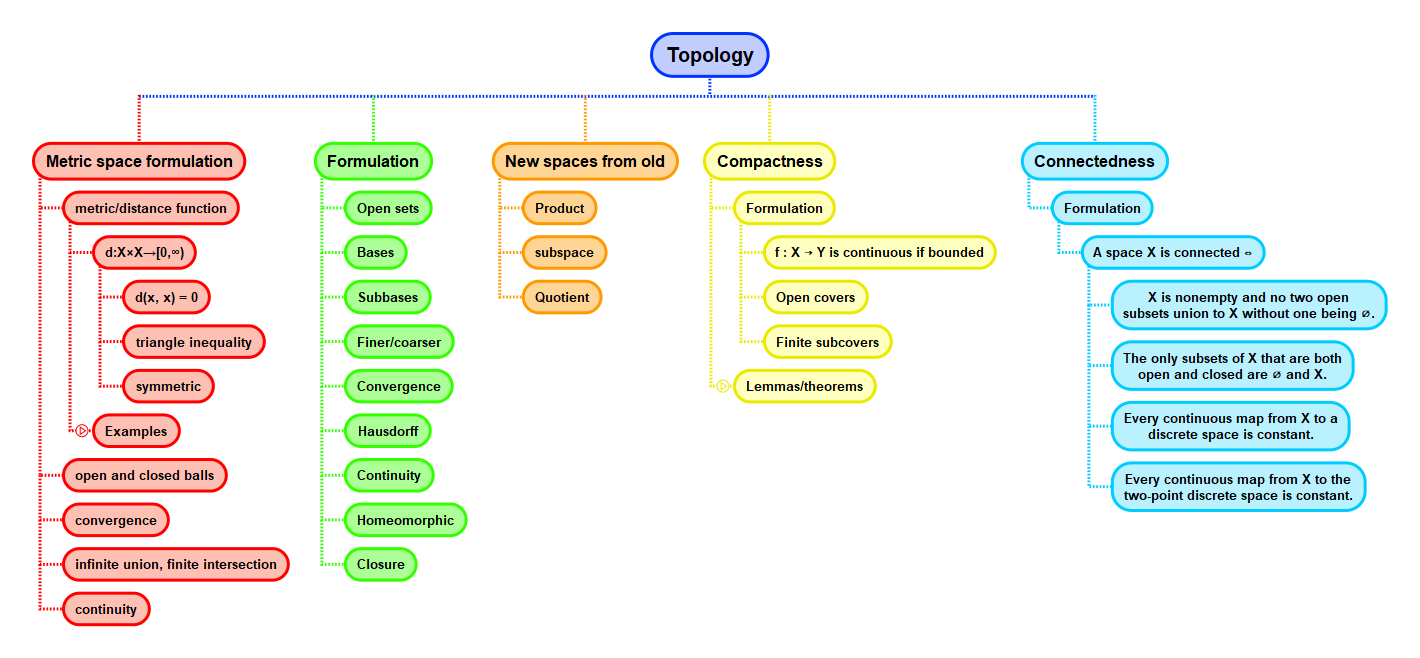Show Question
Math and science::Topology

# Subbasis

Let $$X$$ be a set. A subbasis for a topology on $$X$$ is a collection of subsets of $$X$$ whose union equals $$X$$.

Let $$\mathcal{S}$$ be a subbasis for a topology on $$X$$. The set of all unions of finite intersections of elements of $$\mathcal{S}$$ is a topolgy on $$X$$. This topology is said to be generated by the subbasis $$\mathcal{S}$$.

It can be proved that the generated set forms a valid topology.

### Basis vs subbasis

A subbasis is an even more lightweight description of a topology compared to a basis. A subbasis can generate a basis for the same topology: the collection of all finite intersections of subbasis elements forms a basis.

## Context